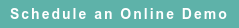# RoyaltyStat Blog

### Posts by Topic

We posit that enterprise profits (P(t) = EBITDA(t)) depend on the same period sales (S(t)) and on previous period sales such that the weights of past period sales decline as a power function. This means that enterprise profits depend on a weighted sum of current and past sales whose parameters we can estimate using the Koyck transform equation:

(1)     P(t) = α + β S(t) + λ P(t – 1) + V(t) ,

where the errors V(t) are subject to an MA(1) process:

(2)     V(t) = U(t) − λ U(t – 1)

and 0 < λ < 1 so the influence of lagged values of sales declines over-time. The variable U(t) is a random shock component.

The short-run profit margin is β and the long-run profit margin (EBITDA Margin) is calculated by setting P(t) = P(t – 1) = μ in Koyck regression equation (1):

(3)     μ = β / (1 – λ).

G. Maddala (1977), Section 9-3 (Lagged Dependent Variables), which includes an explanation of the distinction between short-run and long-run regression coefficients on p. 142; and Section 16-2 (Geometric or Koyck Lag). Our regression equation (1) is equivalent to Maddala's equations 9-7, 9-8, 10-7, and 16-13. Dhrymes (1971), Chapter 5 (Geometric Lag Structure II) contains an ad nauseam treatment of equation (1).

To illustrate, we estimated (1) for nine major US pharmaceuticals MNE (multinational enterprises). The group regression results for Abbott Laboratories (GVKEY 1078), Balchem Corp. (1979), Baxter International (2086), Bristol-Myers Squibb (2403), Eli Lilly (6730), Johnson & Johnson (6266), Merck (7257), Nature's Sunshine (7799), and Pfizer (8530) include for each MNE 40 annual profits disclosed from 1978 to 2018 (we ignore the intercept):

P(t) = 0.2384 S(t) + 0.0657 P(t – 1)

We used Prais-Winsten coefficient estimates, showing similar results as using the Cochrane-Orcutt method. But the OLS estimates from Koyck regression (1) are consistent and their standard errors are asymptotic. For details, see Theil (1971), pp. 260-261, 417.

Consistent means that the probability of the estimated statistics (such as the estimated regression coefficient) is closer to the true value of the parameter when we increase sample size. See Salsburg (2001), Chapter 7 (Fisher Triumphant).

The adjusted R2 = 0.8795 and both regression coefficients are significant at the usual 95% confidence level. The t-value of the lagged dependent variable is 3.215 and the t-value of the exogenous sales variable is 44.565. The autoregressive Prais-Winsten coefficient is ρ = 0.7291 and its t-value is 17.973.

The corresponding long-run profit margin is computed according to (3):

μ = 0.2384 / (1 – 0.0657) = 0.2552 or μ = 25.52%.

We employed 360 observations (40 years of data for each company x 9 companies = 360 observations) that contain no outliers measured by Tukey's filter. Using such full information sample, including all years per MNE profits and sales data available, we obtain similar estimates of the central value of the profit margin applying several statistical methods: WAVG = 24.59%, Tukey's Trimean = 24.59%, Median = 25.46%, Q1 = 18.95%, Q3 = 30.44%, and STDEV = 9.122%. See Tukey (1977), pp. 43, 44, 46.

Of course, innocent use of limited three-year data from selected comparables under the OECD TPG wouldn't enjoy the reliability benefits of full-information large data samples. We used Standard & Poor’s Global Compustat® database of company financials, which we license with distribution rights.

Determining the most reliable measure of arm's length (or comparable) profits is not trivial. This decision requires advanced knowledge of economics and statistics. To be defensible, we must test model (1) against a rival model specification, such as against the OECD simple linear regression model selection (considering the relevant facts and circumstances) to determine what's the most reliable profit indicator to benchmark crossborder controlled transactions.

REFERENCES

Phoebus Dhrymes, Distributed Lags, Holden-Day, 1971.

David Salsburg, The Lady Tasting Tea, A. H. Freeman, 2001.

Henri Theil, Principles of Econometrics, John Wiley & Sons, 1971.

John Tukey, Exploratory Data Analysis, Addison-Wesley, 1977.

Published on Aug 20, 2019 3:03:03 PM

Ednaldo Silva (Ph.D.) is founder and managing director of RoyaltyStat. He helped draft the US transfer pricing regulations and developed the comparable profits method called TNNM by the OECD. He can be contacted at: esilva@royaltystat.com

RoyaltyStat provides premier online databases of royalty rates extracted from unredacted license agreements
and normalized company financials (income statement, balance sheet, cash flow). We provide high-quality data, built-in analytical tools, customer training and attentive technical support.Topics: Net Profit Indicator